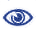# The Prague Stringology Conference 2009

Asymptotic Behaviour of the Maximal Number of Squares in Standard Sturmian Words

 Abstract: Denote by sq(w) the number of distinct squares in a string w and let S be the class of standard Sturmian words. They are generalizations of Fibonacci words and are important in combinatorics on words. For Fibonacci words the asymptotic behaviour of the number of runs and the number of squares is the same. We show that for Sturmian words the situation is quite different. The tight bound 8/10 |w| for the number of runs was given in Baturo (2008). In this paper we show that the tight bound for the maximal number of squares is 9/10 |w|. We use the results of Damanik (2003) where exact (but not closed) complicated formulas were given for sq(w) for w ∈ S and we show: (1) for all w ∈ S  sq(w) ≤ 9/10 |w|+4, (2) there is an infinite sequence of words wk ∈ S such that limk→∞ |wk| = ∞  and  limk→∞ sq(wk) / |wk| = 9/10. Surprisingly the maximal number of runs is reached by the words with recurrences of length only 5. This contrasts with the situation of Fibbonaci words, though standard Sturmian words are natural extension of Fibonacci words. If this length drops to 4, the asymtotic behaviour of the maximal number of squares falls down significantly below 9/10 |w|. The structure of Sturmian words rich in squares has been discovered by us experimentally and verified theoretically. The upper bound is much harder, its proof is not a matter of simple calculations. The summation formulas for the number of squares are complicated, no closed formula is known. Some nontrivial reductions were necessary.

 Download paper:PostScript PDF BibTeX reference
 Download presentation: